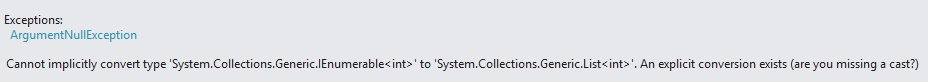How to skip over a number of records using a LINQ query

## Introduction

In order to skip over a number of records in a list of entities with LINQ, we use the Skip method.

## Skip

The Skip method allows you to skip over a specified number of elements in a list and return the remaining elements. The number of elements to skip over is passed as a parameter of the method.

## Examples

#### Simple usage

``````List<int> list = new List<int> { 23, 80, 7, 19, 140, 1009, 30, 2, 14, 6, 97, 450};

List<int> values = list.Skip(8).ToList(); // 14, 6, 97, 450
``````

Note: we use the ToList method in order to avoid the following casting error:#### Usage in Conjunction with the Where method

``````List<int> list = new List<int> { 23, 80, 7, 19, 140, 1009, 30, 2, 14, 6, 97, 450 };

List<int> values = list.Where(l => l > 20).Skip(5).ToList(); // 97, 450
``````

If the parameter passed into the Skip method is greater than the actual size of the list, the result will be an empty list.

``````List<int> list = new List<int> { 23, 80, 7, 19, 140, 1009, 30, 2, 14, 6, 97, 450 };

List<int> values = list.Skip(1000).ToList();  // { }
``````

Besides using primitive types with the Skip method, we can also use reference types.

``````public class Student {

public string FullName { get; set; }

public int Age { get; set; }
}
``````
``````List<Student> students = new List<Student> {
new Student{ FullName = "Emilio Maxwell", Age = 23},
new Student{ FullName = "Shannon Vaughn", Age = 14},
new Student{ FullName = "Emilio Maxwell", Age = 17},
new Student{ FullName = "Alma Cunningham", Age = 15},
new Student{ FullName = "Gilberto Christensen", Age = 20},
new Student{ FullName = "Emmett Banks", Age = 19},
new Student{ FullName = "Robyn May", Age = 16},
new Student{ FullName = "Candice Thompson", Age = 13}
};

List<Student> values = students.Skip(5).ToList();  // { Emmett Banks, Robyn May, Candice Thompson }
``````

## Namespace

To use the Skip method, you have to include this namespace :

``using System.Linq;``